JEE  >  Logarithm: Concepts with Examples

# Logarithm: Concepts with Examples - Notes | Study Physics For JEE - JEE

 Table of contentsIntroduction1 Crore+ students have signed up on EduRev. Have you?

Introduction

In this unit we are going to be looking at logarithms. However, before we can deal with logarithms. we need to revise indices. This is because logarithms and indices are closely related, and in order to understand logarithms a good knowledge of indices is required.
We know that
16 = 24
Here, the number 4 is the power. Sometimes we call it an exponent. Sometimes we call it an index. In the expression 24, the number 2 is called the base.
Example
We know that 64 = 82.
In this example 2 is the power, or exponent, or index. The number 8 is the base.

Why do we study logarithms ?
In order to motivate our study of logarithms, consider the following:
we know that 16 = 24. We also know that 8 = 23
Suppose that we wanted to multiply 16 by 8.
One way is to carry out the multiplication directly using long-multiplication and obtain 128. But this could be long and tedious if the numbers were larger than 8 and 16. Can we do this calculation another way using the powers ? Note that
16 × 8 can be written 24 × 23
This equals  = 27
using the rules of indices which tell us to add the powers 4 and 3 to give the new power, 7. What was a multiplication sum has been reduced to an addition sum. Similarly if we wanted to divide 16 by 8:
16 ÷ 8 can be written 24 ÷ 23
This equals = 2or simply 2
using the rules of indices which tell us to subtract the powers 4 and 3 to give the new power, 1. If we had a look-up table containing powers of 2, it would be straightforward to look up 27 and obtain 27 = 128 as the result of finding 16 × 8.
Notice that by using the powers, we have changed a multiplication problem into one involving addition (the addition of the powers, 4 and 3). Historically, this observation led John Napier (1550-1617) and Henry Briggs (1561-1630) to develop logarithms as a way of replacing multiplication with addition, and also division with subtraction.

What is a logarithm ?

Consider the expression 16 = 24. Remember that 2 is the base, and 4 is the power. An alternative, yet equivalent, way of writing this expression is log2 16 = 4. This is stated as ‘log to base 2 of 16 equals 4’. We see that the logarithm is the same as the power or index in the original expression. It is the base in the original expression which becomes the base of the logarithm.
The two statements

• 16 = 24
• log2 16 = 4

are equivalent statements. If we write either of them, we are automatically implying the other.
Example

• If we write down that 64 = 8then the equivalent statement using logarithms is log8 64 = 2.

Example

• If we write down that log3 27 = 3 then the equivalent statement using powers is 33 = 27.

So the two sets of statements, one involving powers and one involving logarithms are equivalent.

In the general case we have:

Key Point
if x = an then equivalently loga x = n
loga a = 1

Let us develop this a little more.
Because 10 = 101 we can write the equivalent logarithmic form log10 10 = 1.

Similarly, the logarithmic form of the statement 21 = 2 is log2 2 = 1.
In general, for any base a, a = a1 and so loga a = 1
We can see from the Examples above that indices and logarithms are very closely related. In the same way that we have rules or laws of indices, we have laws of logarithms. These are developed in the following sections.

The first law of logarithms

Suppose
x = an and y = am
then the equivalent logarithmic forms are
loga x = n and loga y = m             .....(1)
Using the first rule of indices

xy = an× am = an+m
Now the logarithmic form of the statement xy = an+m is loga xy = n + m. But n = loga x and m = loga y from (1) and so putting these results together we have
loga xy = loga x + loga y
So, if we want to multiply two numbers together and find the logarithm of the result, we can do this by adding together the logarithms of the two numbers. This is the first law.

Key Point
loga xy = loga x + loga y

The second law of logarithms

Suppose x = an, or equivalently loga x = n. Suppose we raise both sides of x = an to the power m:
xm = (an)m
Using the rules of indices we can write this as
xm = anm
Thinking of the quantity xm as a single term, the logarithmic form is
loga xm = nm = mloga x
This is the second law. It states that when finding the logarithm of a power of a number, this can be evaluated by multiplying the logarithm of the number by that power.

Key Point

loga xm = mloga x

The third law of logarithms

As before, suppose
x = an and y = am
with equivalent logarithmic forms
loga x = n and loga y = m       ....(2)
Consider x ÷ y.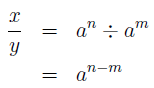using the rules of indices.
In logarithmic form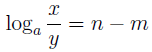which from (2) can be written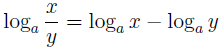This is the third law.

Key Point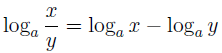The logarithm of 1

Recall that any number raised to the power zero is 1: a0 = 1. The logarithmic form of this is

loga 1 = 0

Key Point
loga 1 = 0

The logarithm of 1 in any base is 0.

Examples

Example 1: Suppose we wish to find log2 512.
This is the same as being asked ‘what is 512 expressed as a power of 2 ?’
Now 512 is in fact 29 and so log2 512 = 9.

Example2: Suppose we wish to find log8 1/64
This is the same as being asked ‘what is 1/64 expressed as a power of 8 ?’
Now 1/64 can be written 64−1. Noting also that 82 = 64 it follows that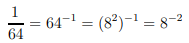using the rules of indices. So log8 = 1/64 = -2

Example3: Suppose we wish to find log5 25.
This is the same as being asked ‘what is 25 expressed as a power of 5 ?’
Now 52 = 25 and so log5 25 = 2.

Example4: Suppose we wish to find log25 5.
This is the same as being asked ‘what is 5 expressed as a power of 25 ?’
We know that 5 is a square root of 25, that is 5 = √25. So 25 1/2 = 5 and so log25 5 = 1/2.

Notice from the last two examples that by interchanging the base and the number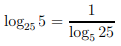This is true more generally:

Key Point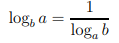Example5: Consider log2 8. We are asking ‘what is 8 expressed as a power of 2 ?’ We know that 8 = 2and so log2 8 = 3.
What about log8 2 ? Now we are asking ‘what is 2 expressed as a power of 8 ?’ Now 23 = 8 and so 2 = ∛8 or 81/3. So log8 2 =1/3.

We see again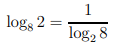Standard bases

There are two bases which are used much more commonly than any others and deserve special mention. These are
base 10 and base e

Logarithms to base 10, log10, are often written simply as log without explicitly writing a base down. So if you see an expression like log x you can assume the base is 10. Your calculator will be pre-programmed to evaluate logarithms to base 10. Look for the button marked log.
The second common base is e. The symbol e is called the exponential constant and has a value approximately equal to 2.718. This is a number like π in the sense that it has an infinite decimal expansion. Base e is used because this constant occurs frequently in the mathematical modelling of many physical, biological and economic applications. Logarithms to base e, loge, are often written simply as ln. If you see an expression like ln x you can assume the base is e. Such logarithms are also called Naperian or natural logarithms. Your calculator will be pre-programmed to evaluate logarithms to base e. Look for the button marked ln.

Key Point
Common bases: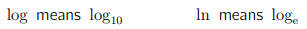where e is the exponential constant.

Useful results:

log 10 = 1, ln e = 1

Using logarithms to solve equations

We can use logarithms to solve equations where the unknown is in the power. Suppose we wish to solve the equation 3x = 5. We can solve this by taking logarithms of both sides. Whilst logarithms to any base can be used, it is common practice to use base 10, as these are readily available on your calculator. So, log 3x = log 5
Now using the laws of logarithms, the left hand side can be re-written to give
x log 3 = log 5
This is more straight forward. The unknown is no longer in the power. Straightaway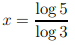If we wanted, this value can be found from a calculator.

The document Logarithm: Concepts with Examples - Notes | Study Physics For JEE - JEE is a part of the JEE Course Physics For JEE.
All you need of JEE at this link: JEE

## Physics For JEE

257 videos|633 docs|256 tests
 Use Code STAYHOME200 and get INR 200 additional OFF

## Physics For JEE

257 videos|633 docs|256 tests

Track your progress, build streaks, highlight & save important lessons and more!

,

,

,

,

,

,

,

,

,

,

,

,

,

,

,

,

,

,

,

,

,

;